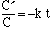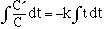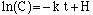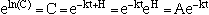Name: Catherine Sullivan Who is asking: Student Level: Secondary Question: Please help me with the following: The radioactive isotope carbon-14 is present in small quantities in all life forms, and it is constantly replenished until the organism dies, after which it decays to carbon-12 at a rate proportional to the amount of C-14 present, with a half life of 5730 years. Suppose C(t) is the amount of C-14 at time t. Find the value of the constant k in the differential equation: C'=-kC In 1988 3 teams of scientists found that the Shroud of Turin, which was reputed to be the burial cloth of Jesus, contained 91% of the amount of C-14 contained in freshly made cloth of the same material. How old is the Shroud according to the data? Note: for part 1, I know how to solve for k using the equation C=C*ekt, but I need to do it from the differential equation. I took the integral of both sides but was left with a first derivative and too many unknowns. Please help!! Thanks Hi Catherine, C is the amount of carbon-14 that is present at time t. (Sometimes it helps to write C(t) to emphasize that it is a function of t.) Start with the differential equation C' = -k C and divide both sides by C to getIntegrate both sides with respect to t and the equation becomesIntegration produceswhere H is a constant. Now solve for C by taking the expontanial of each side and you getwhere A is the constant eH. Thus you have the equation C = A e-k t which you can solve for k. Cheers, Harley Go to Math Central